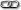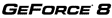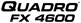Developer Site Homepage Developer News HomepageDeveloper Login Become aRegistered DeveloperDeveloper Tools Documentation DirectX OpenGL GPU Computing Handheld Events CalendarNewsletter Sign-Up Drivers Jobs (1) Contact Legal InformationSite Feedback## NVIDIA CUDA SDK - Data-Parallel Algorithms

The CUDA Developer SDK provides examples with source code, utilities, and white papers to help you get started writing software with CUDA. The SDK includes dozens of code samples covering a wide range of applications including:

• Simple techniques such as C++ code integration and efficient loading of custom datatypes
• How-To examples covering CUDA BLAS and FFT libraries, texture fetching in CUDA, and CUDA interoperation with the OpenGL and Direct3D graphics APIS
• Linear algebra primitives such as matrix transpose and matrix-matrix multiplication
• Data-parallel algorithms such as parallel prefix sum of large arrays
• Performance: profiling using timers and bandwidth tests
• Advanced application examples such as image convolution, Black-Scholes options pricing and binomial options pricing
 256-bin HistogramThis sample demonstrates efficient implementation of 256-bin histogram.or later 64-bin HistogramThis sample demonstrates efficient implementation of 64-bin histogram.or later Separable ConvolutionThis sample implements a separable convolution filter of a 2D signal with a gaussian kernel.or later Texture-based Separable ConvolutionTexture-based implementation of a separable 2D convolution with a gaussian kernel. Used for performance comparison against convolutionSeparable.or later Bitonic SortBitonic sort is a very simple parallel sorting algorithm that is very efficient when sorting a small number of elements: http://citeseer.ist.psu.edu/blelloch98experimental.html This implementation is based on: http://www.tools-of-computing.com/tc/CS/Sorts/bitonic_sort.htmor later N-Body SimulationThis sample demonstrates efficient all-pairs simulation of a gravitational n-body simulation in CUDA. This sample accompanies the GPU Gems 3 chapter "Fast N-Body Simulation with CUDA".or later Parallel ReductionA parallel sum reduction that computes the sum of large arrays of values. This sample demonstrates several important optimization stratezies for parallel algorithms like reduction.or later This sample uses CUDA to compute and display the Mandelbrot set.or later Fast Walsh TransformNaturally(Hadamard)-ordered Fast Walsh Tranform for batched vectors of arbitrary eligible(power of two) lengthsor later This example demonstrates an efficient CUDA implementation of parallel prefix sum, also known as "scan". Given an array of numbers, scan computes a new array in which each element is the sum of all the elements before it in the input array.or later Scan of Large ArraysThis example demonstrates an efficient CUDA implementation of parallel prefix sum (also known as "scan") for arbitrary-sized arrays. Given an array of numbers, scan computes a new array in which each element is the sum of all the elements before it in the input array.or later